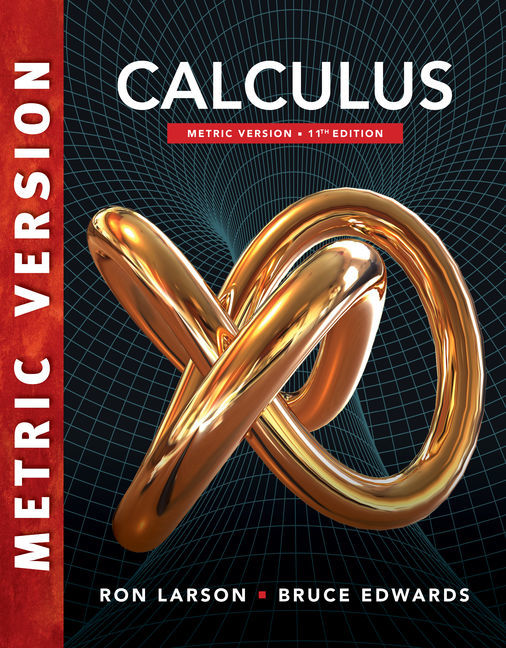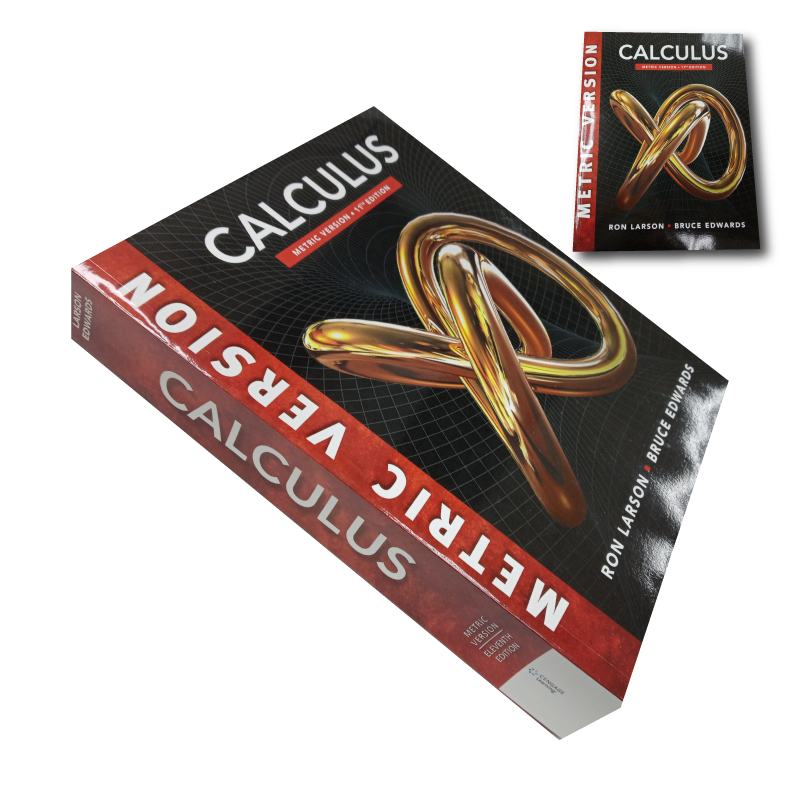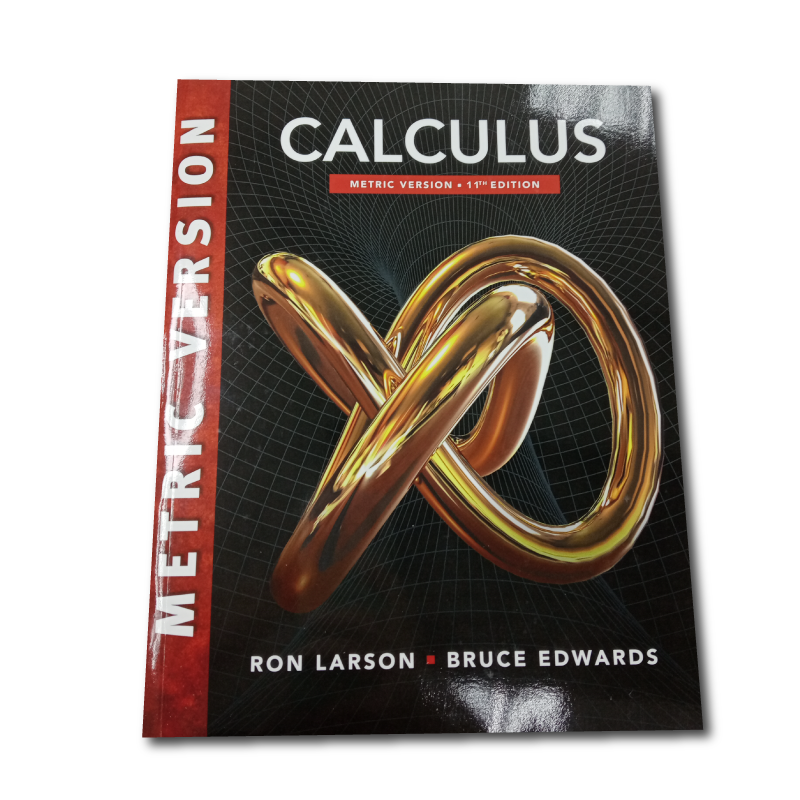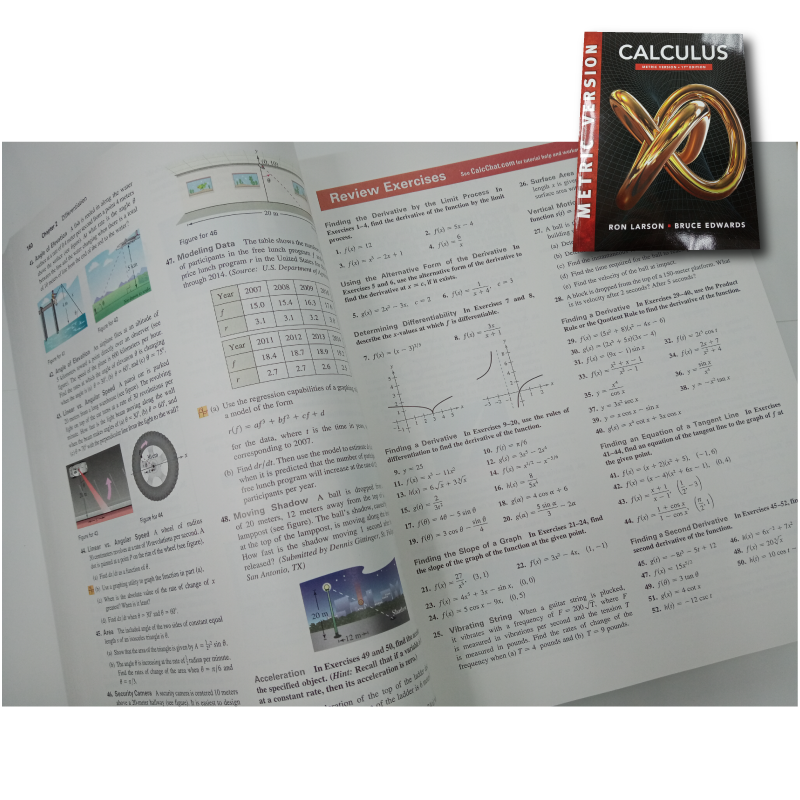••••# Calculus, Metric Version, 11th Edition

RM 125.00
- +

RON LARSON | BRUCE EDWARDS

9781337616195

With a long history of innovation in the market, Larson/Edwards’ CALCULUS, INTERNATIONAL METRIC EDITION has been widely praised by a generation of students and professors for solid and effective pedagogy that addresses the needs of a broad range of teaching and learning styles and environments. Each title in the series is one component in a comprehensive calculus course program that carefully integrates and coordinates print, media, and technology products for successful teaching and learning

P. PREPARATION FOR CALCULUS.
1. LIMITS AND THEIR PROPERTIES.
2. DIFFERENTIATION.
3. APPLICATIONS OF DIFFERENTIATION.
4. INTEGRATION.
5. LOGARITHMIC, EXPONENTIAL, AND OTHER TRANSCENDENTAL FUNCTIONS.
6. DIFFERENTIAL EQUATIONS.
7. APPLICATIONS OF INTEGRATION.
8. INTEGRATION TECHNIQUES AND IMPROPER INTEGRALS.
9. INFINITE SERIES.
10. CONICS, PARAMETRIC EQUATIONS, AND POLAR COORDINATES.
11. VECTORS AND THE GEOMETRY OF SPACE.
12. VECTOR-VALUED FUNCTIONS.
13. FUNCTIONS OF SEVERAL VARIABLES.
14. MULTIPLE INTEGRATION.
15. VECTOR ANALYSIS.
16. SECOND ORDER DIFFERENTIAL EQUATIONS* ONLINE.
APPENDIX.
A. Proofs of Selected Theorems.
B. Integration Tables.
C. Precalculus Review (Web).
C.1. Real Numbers and the Real Number Line.
C.2. The Cartesian Plane.
D. Rotation and the General Second-Degree Equation (Web).
E. Complex Numbers (Web).
F. Business and Economic Applications (Web).
G. Fitting Models to Data (Web)